## Monday, November 12, 2018

### Chemical Notation with Polyatomic Ions

General Chemistry Index

Where are we going with this? This page will give the ability to demonstrate an understanding of the law of conservation of mass through the use of particle diagrams and mathematical models.

When working with chemical notation, it has been established that the subscripts (or numbers FOLLOWING the elements) represent the number of those elements present. The coefficient tells how many of the molecule is present.

Thus,

3 H20 or 3 H2O or even 3H20

means 3 molecules of water in which are 2 atoms of hydrogen and 1 atom of oxygen.

There is another variation of this notation that is applied in certain cases with some compounds. Because of how the compounds form, there is sometimes a value in keeping some of the elements as a unit and subscripting the whole unit to show how many of that unit are present.

Look at the reaction below:

CaC2   +   2 H2O   --->   Ca(OH)2   +   C2H2

Notice on the product side, the OH is inside parenthesis. This represents that that is a unit of molecules that are being kept together based on how the compound is formed. The subscript indicates that there are two of these units present.

Examples:

3 Ca(OH)2 has in it, 3 Ca, 6 O, and 6 H. (The subscripted 2 applies to both atoms inside the parenthesis, and the coefficient of 3 applies to the whole molecule.)

Ca3(PO4)2 has in it, 3 Ca, 2 P, and 8 O. (The subscripted 2 applies to the PO4, so there are 2 P and 8 0)

2 Cu(NO3)has—to begin with, there are 2 molecules of Cu(NO3)2 as indicated by the coefficient.
• EACH molecule has 1 Cu and 2 (NO3). Since there are 2 (NO3) (The subscript 2 applies to everything inside the parenthesis.), that means there are 2 N and 6 O in each Cu(NO3)molecule.
• Since there are 2 molecules of Cu(NO3)2, there are in TOTAL:

• Cu
• N
• 12 O

Summary:
• Coefficients, the numbers in front, apply to the whole molecule and tell how many molecules or "sets" of molecules are present.
• Subscripts (or number FOLLOWING the atom symbols) tell how many of that atom are in the molecule.
• If a group of atoms are inside parenthesis:

• They are to be kept together as a unit.
• Any subscripts to the closing parenthesis means that there are that many units of the atoms inside the parenthesis are present.

## Wednesday, September 26, 2018

### Meet The ∆ — The Scientific Symbol For Change

Many times in science (and engineering), it is necessary to measure changes in things. There are many examples of this ranging from objects moving or the pressure of gases going from low to high.

The normal way of discussing these changes is to consider initial and final instances. Consider this example:

A runner begins moving at a distance of 20 meters from the picnic table. A moment later the runner is 50 meters from the picnic table. This means there was a change in distance from the picnic table of 30 meters.
In this case:
The initial distance (from the picnic table) is 20 meters
The final distance from the picnic table is 50 meters
The change in distance is 30 meters.
Whereas in science and engineering, using a few numbers and letters as possible is the norm, and whereas different measures have "normal" symbols, the above way of describing the situation is never used in the actual solution of a problem.

The normal symbol for distance is d (but sometimes s for reasons I cannot remember), so it would be expected to use the d for the various distances. But, how can you tell WHICH of the distances being referenced? There must be some other means.

There is.

There are two common ways to distinguish between various distances (or anything else) in the same discussion (a lab writeup, a word problem, etc.). Both ways rely on putting a subscript after the variable symbol.

That will look like this:
VariableSymbolSubscript
So if there are more than once distance, one way is to use numbers for the different distances. Distance 1… Distance 2… Distance 3… That makes sense.

Example time:

A park has three water fountains. One is 20 meters from the pavilion. Another is 150 meters from the pavilion, The last one is 285 meters from the pavilion. Therefore…
d1 = 20 m
d2 = 150 m
d3 = 285 m
Another way to distinguish between different measures of the same quantity is to look at the "before and after" information. In such a case, the idea if "initial" and "final" are represented by the subscripts of i and f.

The usual symbol for temperature is T…

Example time:

A ball is kept in a refrigerator where the temperature is 10 C. It is moved out into the room where the temperature is 23 C.
Therefore…
Ti = 10 C
Tf = 23 C
It is not unheard of to use 1 and 2 as the subscripts, even when it is clearly a before and after situation. Thus, the above information could be written as:
T= 10 C
T2 = 23 C

INTRODUCING THE ∆

In many, many cases, the most interesting part of a situation is how the variable changes. In science and engineering, the change in something is represented by the  symbol before the normal quantity symbol. Therefore, given the typical symbols for distance and temperature:

The amount that distance changed would be written as ∆d
and
the amount that temperature changed would be written as ∆T.

The concept of change is not limited to distance or temperature. Any measured quantity works. If something changes value, the ∆ can be used to represent the change.

Some "Change" Words

In stories or word problems, some words strongly represent a change in the measure. When encountered, these words will correspond to the ∆. This is NOT EVERY way to indicate use of the ∆, but usually, when you see these words, it will be not the initial or final value, but the ∆ value:
increased by
decreased by
changed by
moved
farther
closer
more

Calculating Change

Finding the change in something is really easy. How much did you start with? How much did you end up with? What's the difference.

It works the same way with distance, pressure, velocity, time, temperature, volume… it works the same with any measured quantity.

As a formula, if you are looking at distance, it looks like this.

∆d = df - di

NOTE: if you are using 1s and 2s as the subscripts, you need to make sure you know which was the final and which were the initial values.

SUMMARY

1. When more than one measure of the same quantity is required, the different measures are indicated by subscripts.

2. Subscripts can be i and f for initial and final, or can be numbers representing different measures.

3. The change in the measured thing is represented by the ∆ followed by the normal symbol.

## Friday, May 4, 2018

### Curving pitches, kicks, and shots…

So… In baseball and softball, many batters batters hate the curveball… Because it curves…
NOTE: The physics of this is true for ALL sports where a ball moves through the air… tennis, golf, ping pong, volleyball, soccer (bend it like Becker)…
Now, the curve in question is in addition to the fact that it is moving from the picture to the batter as a projectile which automatically means that it's up and down is changing at the same time it moves closer and closer to the batter.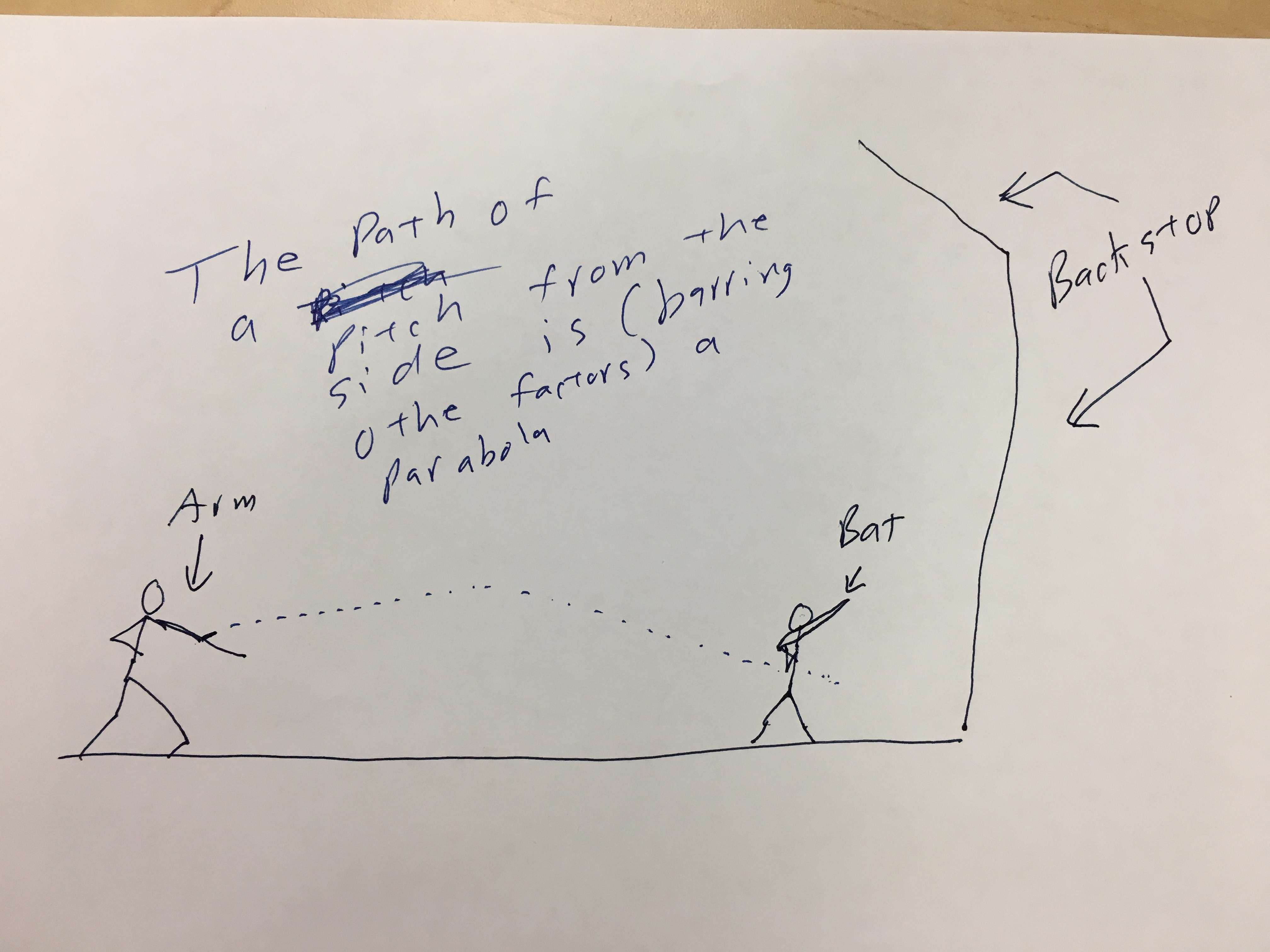Imagine slow-pitch softball or a lobbed pitch… In the extreme, think about soft toss… The ball moves up and down as it approaches the strike zone.
So, every pitch regardless of type is, from the moment it is released affected by these three forces:
1. Gravity pulls the ball down toward the center of the earth.
2. There is a tiny, tiny buoyant force that is far far less than the force of gravity.
3. There is air resistance (friction) that opposes the motion of they ball and which is always directly directly opposite the direction of the instantaneous velocity.
Now, let's talk about rotation! Because, except for a knuckle ball, pitches rotate…
I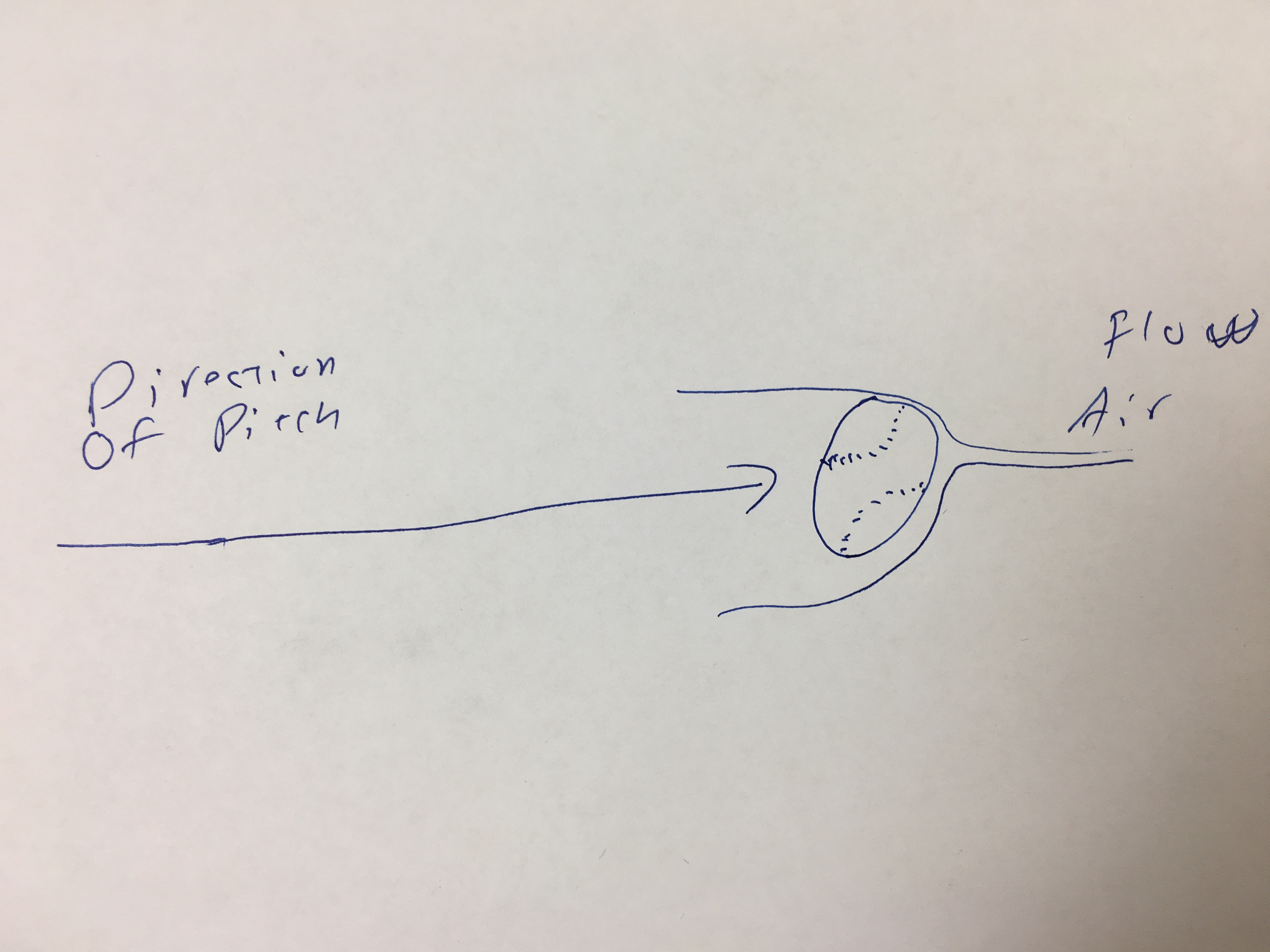magine a ball spinning on an axil, not moving. When the ball spins, the air around it is "drug" in the direction of the spin. Now, if you add in the movement of the ball, something happens!
First if the ball is thrown and spin is ignored, you get an object moving through the air. The air flows around the ball equally. Nothing interesting there…
However, if you account for the spinning of the ball… and the air that is being drug around it by the spin, you start to see something.

When air moves faster, is gets stretched out. There are fewer molecules of air in the same space. From the gas laws, it is known that if you have fewer molecules in the same space, the pressure is lower (given constant temperature, which can be assumed over the diameter of a baseball or softball).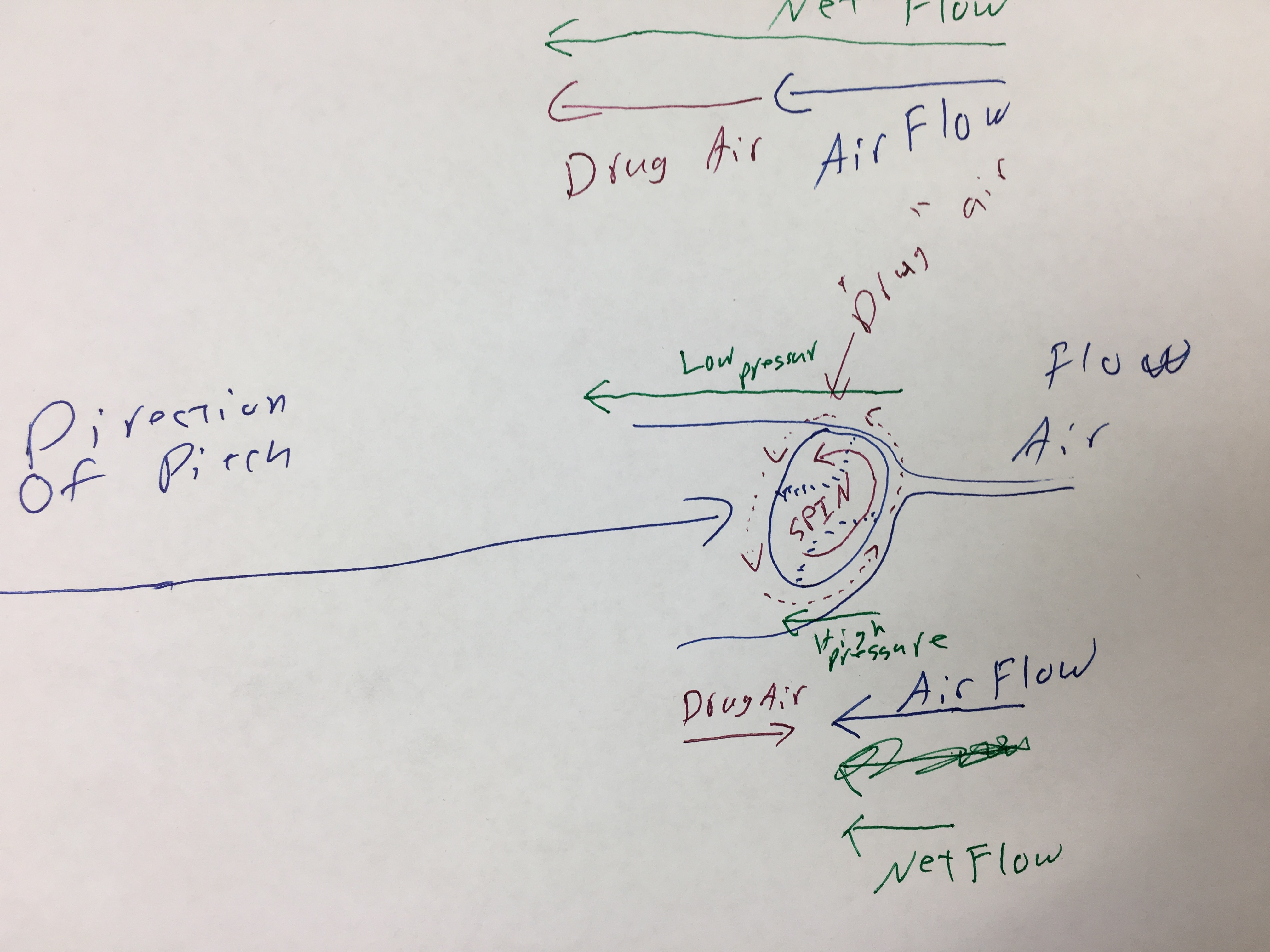So, the spinning ball drags the air around it, AND those moving molecules of air interact with the moving air as the pitch moves through the air.  Where the drug air is going the same way as the air flow, the molecules move faster, but where the drug air is going in the opposite direction, the air flow slows down.
The result is that one side of the ball has high pressure and the other side has low pressure. The difference in pressure creates a force. The air pressure difference pushes the ball form the high pressure region to the low pressure region.
That is to say there is a force acting in the direction of the low pressure. Given Newton's Second Law, it is known that F=ma, so where there is a net force, the ball must accelerate (change velocity) in the direction of the force.
Thus, the rate of acceleration can be found based on some function of the difference in pressure (F) divided by the mass of the ball:
a = ƒ(p1 and p2)/m
So, what can a pitcher do to make the ball curve? Well… you can't control the mass of the ball. You can control, however the spin.
Since the air moving around the ball due to it moving is the same on all sides, the spin accounts for the difference in pressure. Both p1 and p2 are affected by the spin
T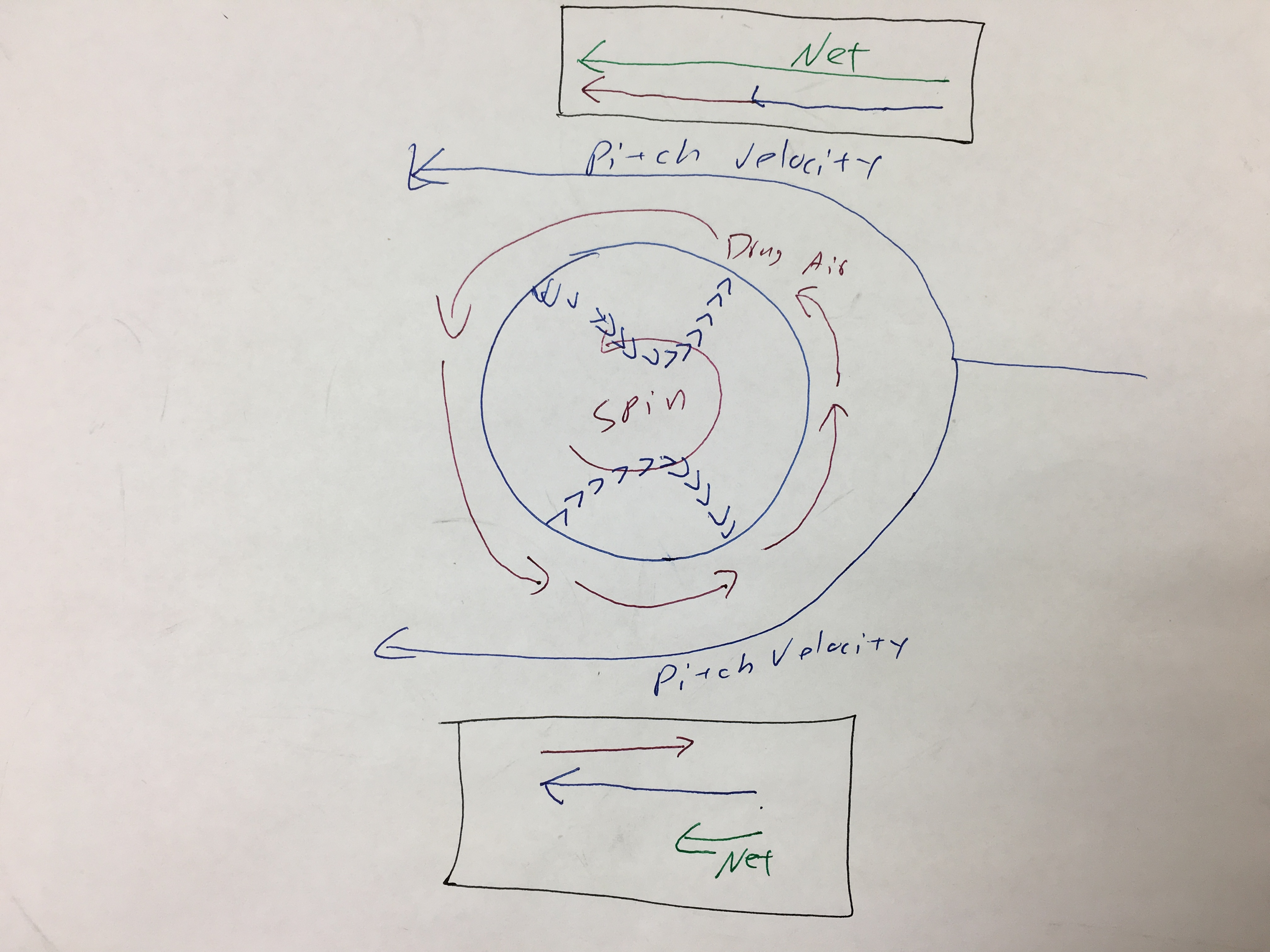he net air flow is on one side pitch velocity + drug air.
The net air flow on the other side is pitch velocity - drug air.
Thus, since the difference in pressure is a result of the DIFFERENCE in the net velocities of the air on opposite sides of the pitch path, the more spin, the bigger the difference in pressure.
AND, then, the bigger the difference in pressure (back to the math)…
a = ƒ(p1 and p2)/m
…the greater the rate of acceleration.
By controlling the spin allows a pitcher to control where the high and low pressure zones are. The ball will break into the low pressure zone.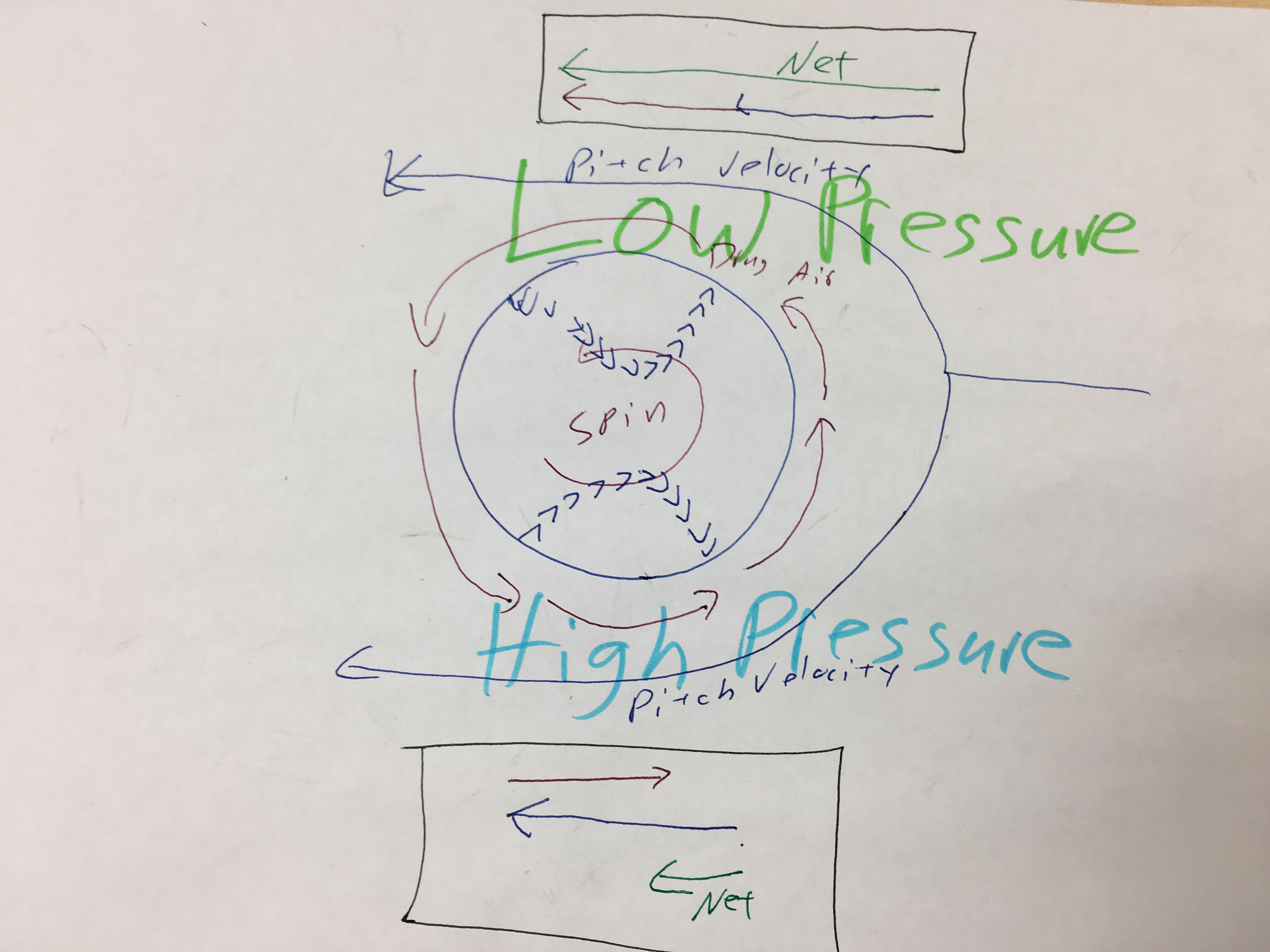A pitch, then, with backspin, will dive. If the rotation is the other way (e.g. a good overhand throw), the ball will actually float some and the effect of gravity will be lessoned.
The LESS the axis of rotation aligns with the path of the ball, the GREATER the affect of the spin on the ball's path.
Conversely, if the axis of rotation aligns with the path of the ball's movement, then the affect of the spin is zero. Think of a spiral football pass. IF the pass/punt spirals properly, the affect of the spin is zero.
An overhand fastball rotates back toward the pitcher. Thus, there is low pressure on top of the ball.
An underhand fastball (or a topspin tennis shot) rotates forward toward the batter. Thus, there is low pressure on the bottom of the ball.
Cutters, sliders, etc have various spins that move the low pressure zone to different places on the ball.
CONCLUSION:
1.) Since the rate at which a ball's velocity changes can be found by
a = ƒ(p1 and p2)/m
and since the difference in pressures is caused by the spin, then changing the direction and rate of the spin will change the direction and rate of the acceleration (of the break).
2.) Increasing the rate of spin increases the difference in pressures. The greater the difference in pressure, the more the ball with break (accelerate).

1.) The diameter of the ball affects the velocity of the spinning surface. Thus, two balls of the same mass spinning at the same rate, but having different diameters will break differently. For example, a huge beachball with the same mass as a volleyball will curve more with the same spin.

## Tuesday, May 1, 2018

### Friction: A Force That Opposes Motion

Newton's Second Law leads to the formula that connects force, mass, and acceleration:

F = ma

Earlier, it was explained that there are only four fundamental forces, but that every other force that is easily observed is derived from them. Thus, friction can be understood as a force which arises due to the interaction of the atoms between two objects when they are in contact. The strong, weak, and electromagnetic forces work such that there is a resistive force when one object slides across another.

A resistive force (also know as oppositional force) works in the opposite direction of the active forces, such as pushing or pulling something.

Before working with friction, there are two more concepts that need to be understood:

### Weight

Weight is the force created by gravity and its magnitude can be found by multiplying the mass of an object by the rate at which gravity accelerates things. On earth, for introductory physics students,. the value for acceleration due to gravity of 9.8 (m/s)/s or 9.81 (m/s)/s is commonly used.

In the metric system, the Newton is the unit for weight. The pound is also a unit of weight*.

The direction for weight is always "down." That means that (when on earth) weight always acts in direction that is perpendicular to the horizon and toward the center of the earth.

### Normal ForceThe concept of Normal Force is easy to understand. Calculating the normal force (which is usually indicated as Fn) requires using trigonometry EXCEPT when the surface on which an object slides is level (has an angle to the horizon of zero).

The formal force (Fn) is defined as the component of the weight (mg) of an object that is perpendicular to a surface. When the surface is level ALL of the normal force is perpendicular to the surface. That makes things easy. Thus, the normal force IS THE WEIGHT, but this is ONLY true with the surface is level (has and angle to the horizon of zero).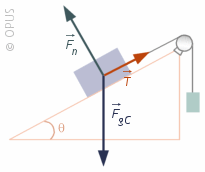When the surface is NOT level, then, math…

Fn is labeled, and it is clear that it pushing the box in a direction that is perpendicular to the surface of the inclined plane.

### Note that F3 is perpendicular to the horizon. That would mean that F3 is the weight or is found as m•g where m is the mass and g is acceleration due to gravity.Force of Friction

There are two types of friction. One is when an object is already sliding. One is when the object is at rest. They are cleverly named sliding friction and static friction.

Sliding friction is sometimes indicated as fk where the k stands for kinetic (which means moving) and static friction is indicated as fs.

NOTE: in this nomenclature, the fi is lowercase. the k and the s may or may not be subscripted, depending on the type-setting process.

Intuition will tell you that pushing something over ice is easier than pushing it over asphalt. There therefore must be some way to indicate the degree of slipperiness an object has (or stickiness).

There is. It is called the coefficient of friction. And there are two sets of coefficients: one for each type of friction. Sliding and static coefficients of friction are abbreviated as µk and µs respectively.

Here is a link that will allow you to look at coefficients of friction for various materials:

https://www.engineeringtoolbox.com/friction-coefficients-d_778.html

### Calculating the Force of Friction

So, using all those concepts, calculating the force of friction becomes pretty simple. Friction can be found by multiplying the normal force by the coefficient of friction:

fk = (Fn)(µk)

EXAMPLE

Clean dry steel sliding on steel has a coefficient of friction of μ = 0.78. If a block with a mass of 4 kg slides across, what is the force of friction.

First, calculate the normal force:

Fn = mg
Fn = (4)(9.8)
Fn = 39.2

Now, use Fn to find the force of friction.

fk = (Fn)(µk)
fk = 39.2 • 0.78

fk = 30.576 N

_________________
*The English system unit for mass is called the Slug and is approximated by taking the weight of something and dividing by 32. So a 110 pound person would have a mass of 3.4 slugs.

## Monday, April 16, 2018

### Finding Time Using the Distance Equation

Any calculation of time (t) is relatively easy in the cases where either vi or a was zero. In the cases where neither are zero, the math results in a second degree polynomial equation such as:
0 = x2 + 3x - 12
Where this occurs in physics of motion is in the full distance equation:
df = di + (vi)(t) + (1/2)(a)(t2)
Although this does not exactly match the expected form for a quadratic equation, it can easily be rearranged as such:

df = di + (vi)(t) + (1/2)(a)(t2)
0 = di - df + (vi)(t) + (1/2)(a)(t2)
0 = (1/2)(a)(t2) + (vi)(t) + di - df

Keep in mind that di - df will yield a number when the values are plugged in and simplified.
To solve these problems, the steps are the same for any problem in science, BUT the algebra becomes harder.
After you have plugged in the numbers, combine like terms and simplify. Suppose the following:
df = 89
di = 50
vi = 4
a = 6
and you need to find t
df = di + (vi)(t) + (1/2)(a)(t2)
89 = 50 + (4)(t) + 1/2(6)(t2)
0 = -39 + 4t + 3t2
(Put it in normal quadratic form.)
0 = 3t2 + 4t - 39
Now you can either factor or use the quadratic formula to find the values for t:

0 = ( t  - 3 )( 3t + 13)
0 = t - 3    AND   0 = 3t + 13
3 = t        AND   -13/3 = t

Since time cannot be negative within the context of classical physics, only t = 3 is a valid answer.
Using the quadratic equation will yield the same results.

While it is far easier to find t when either a or vi is zero, the math to find t when that is not the case is not beyond the skills of a student taking an introductory physics class.
For another look at this process, check out this video:

## Thursday, March 22, 2018

### Physics of Motion: What is What?

Physics of Motion: What is What?

The equations:

df = di + (vi)(t) + (½)(a)(t2)   This will answer almost all “how far” questions.
Use this if:
Only 1 velocity is given
You have a distance given (df is rarely 0, but sometimes it is the unknown)

vf = vi + (a)(t)    This will answer almost all “how fast” questions.
Use this if:
2 velocities are given
You don’t have distance

v(ave) = (vi + vf)/2 (See * below)
Use this if:
You need to find the average velocity
You know BOTH vi and vf (you might have to calculate vf!

vf = (vi + ∆v)
Use this if:
You need to find the final velocity AND
You know BOTH vi and how much v changes (e.g. speed increases by 10 m/s)

df = (di + ∆d)
Use this if:
You need to find the final distance, total distance, or final position AND
You know BOTH di and how much d changes (e.g. it moves 10 meters)

The hints and helps:

It is vi if…
“…traveling at a rate of…”
“…moving at…”
"…has a velocity of…"

If it is “at rest” then vi = 0 and di is probably 0

The words "begin" and "starts" generally go with the initial values.

If it is “moving at a constant” rate or if it “has a constant velocity” then a = 0

It is possible that something not given (but which is not the thing to be found) should have a value of 0...

*Another v(ave) approach:

v(ave) = ((vi + at) + vi)/2
Use this if: You need to find the average velocity You know vi and but you have to calculate vf